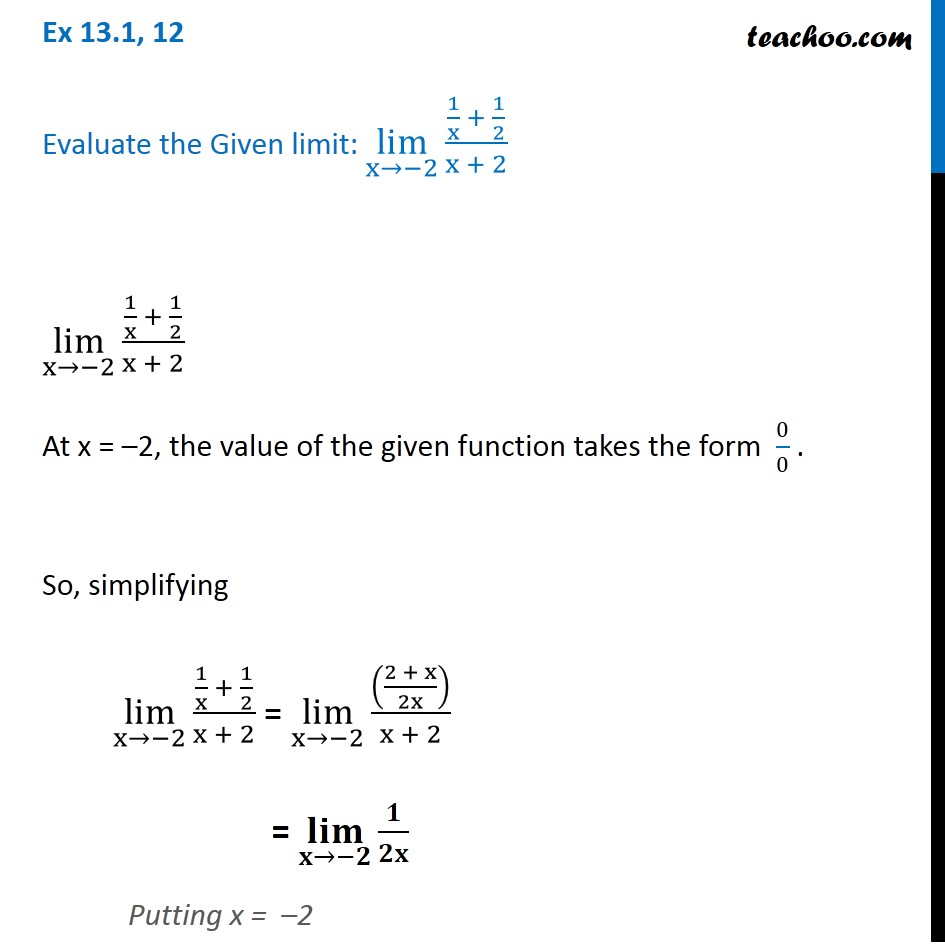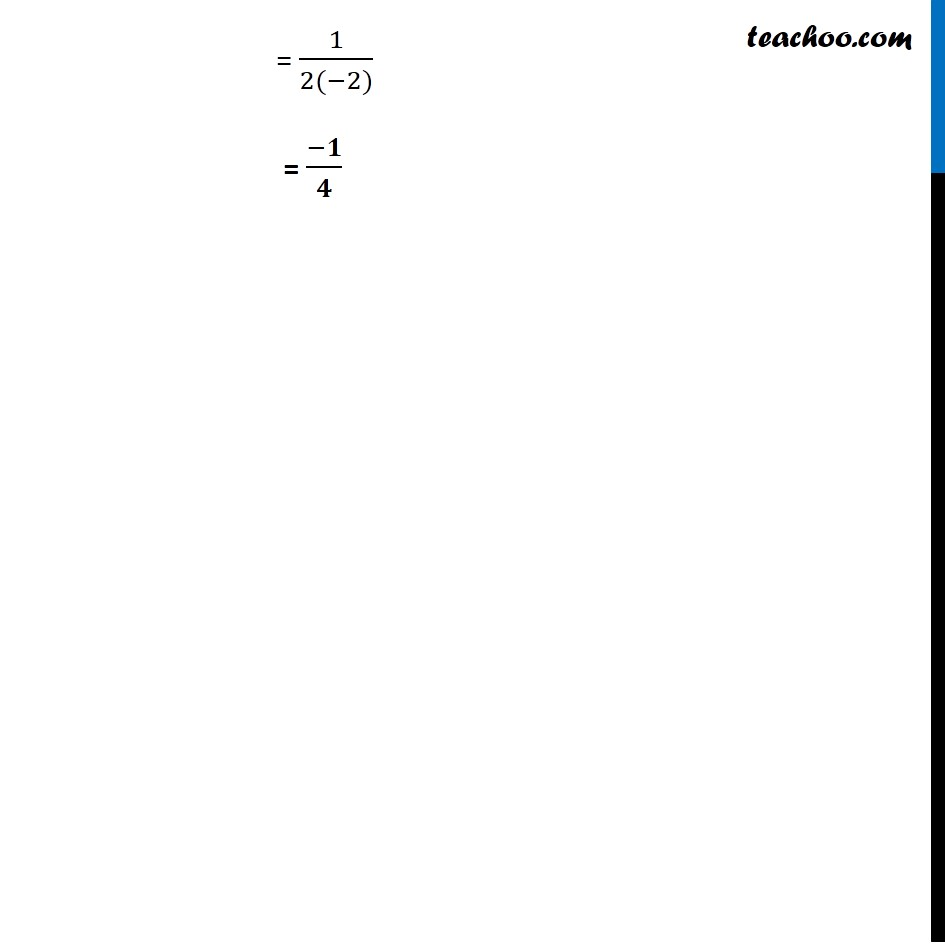Ex 13.1 (Term 1)

Chapter 13 Class 11 Limits and Derivatives (Term 1 and Term 2)
Serial order wise### Transcript

Ex 13.1, 12 Evaluate the Given limit: lim┬(x→−2) (1/x + 1/2)/(x + 2) lim┬(x→−2) (1/x + 1/2)/(x + 2) At x = –2, the value of the given function takes the form 0/0 . So, simplifying lim┬(x→−2) (1/x + 1/2)/(x + 2) = lim┬(x→−2) (((2 + x)/2x))/(x + 2) = (𝐥𝐢𝐦)┬(𝐱→−𝟐) 𝟏/𝟐𝐱 Putting x = –2 = 1/(2(−2)) = (−𝟏)/𝟒